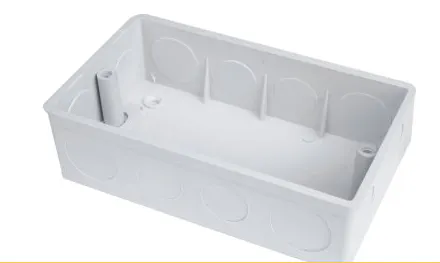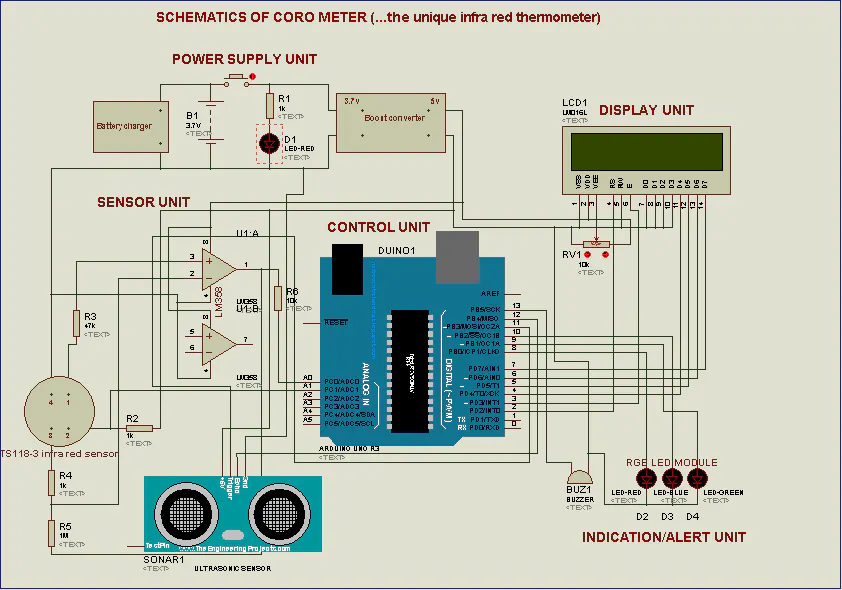# "CORO METER" (... the unique infra red thermometer)

This device measures body temperature. From 38 degree Celsius upwards, the device triggers alarm and displays emergency number(s) to call.

BeginnerFull instructions provided2 hours454

## Things used in this project

### Hardware components

 Male-male jumper wires set
×1
 Female-female jumper wire (set)
×1
 Buzzer 3V-24V (Active Buzzer)
×1
 RGB LED module
×1
 Switch
×1
 104 0.1uf 100nf 100000pf ceramic capacitor
×1
 1K resistor
×3
 LM358
×1
 Battery charger
×1
 3.7v battery holder
×1
 18650 3.7v 3000mah
×1
 TS118-3 non contact infrared temperature sensor
×1
×1
 47KΩ 1/4W resistor
×1
 8.2KΩ 1/4W resistor
×2
 68K resistor
×1
 vero board big size dotted
×1
×1
 10KΩ Potentiometer
×1
 Blue LCD 1602
×1
 Arduino Nano V3.0
×1

### Software apps and online servicesProteus Professional

### Hand tools and fabrication machines

 PCB hand drilling set
 Screw driver set 6032A
 DT830D multimeter
 DT830D multimeter
 Professional soldering iron 60W
 60watts Hot melt glue gun

## Custom parts and enclosures

You can get it here: https://flutterwave.com/store/evatronics-ffv7sbfpqpeu?_ga=2.221296977.811489624.1593877687-906788748.1592644606## Schematics

### SCHEMATICS OF CORO METER (...the unique infra red thermometer)

Carefully study this design, gather the components, implement, test, package and roll out.## Code

### SOURCE CODE FOR "CORO METER" (...the unique infra red thermometer)

Arduino
This source code is a .ino file. Install arduino IDE from www.arduino.cc, open this source code with the IDE, update your libraries if any is missing, compile the source code and upload it to your arduino nano.
```#include <Wire.h>
#include <LiquidCrystal.h>
LiquidCrystal lcd (2,3,4,5,6,7);

#define trigPin 11
#define echoPin 12
#define redLight 8
#define blueLight 10
#define greenLight 9
#define buzzerPin 13
char degree = 223;

long duration, distance;
float objtemp,ambtemp;

// Declaring pins...

#define THERMOPILE A0
#define THERMISTOR A1

// Declaring constants for the thermistor calculations...

const float a = -412.6;
const float b = 140.41;
const float c = 0.00764;
const float d = -0.0000000000000000625;
const float e = -0.00000000000000000000000115;

// Declaring constants for the thermopile calculation...

const float k = 0.004313;
const float delta = 2.468;

const float reftemp = 25; // 25 degree Celsius used as reference temperature
const float shiftv = 0.6; // Degree of shift for thermopile voltage for negative V values in range
const float verr = 0.6;  // voltage error introduced to thermopile by circuit

void setup()
{
Serial.begin(9600);
pinMode(trigPin, OUTPUT);
pinMode(echoPin, INPUT);
pinMode(buzzerPin, OUTPUT);
lcd.begin(16,2);
lcd.setCursor(0,0);
lcd.print("IR THERMOMETER");
lcd.setCursor(2,1);
lcd.print("( CORO METER )");
delay(2000);
lcd.clear();
lcd.setCursor(0,0);
lcd.setCursor(0,1);
lcd.print("by: EVATRONICS");
delay(2000);
lcd.clear();
}

void loop()
{
measureTemperature();
checkDistance();

if(distance<=60)
{
if(objtemp<38)
{
digitalWrite(greenLight,LOW);
digitalWrite(blueLight,HIGH);
lcd.setCursor(0,0);
lcd.print("Temp: ");
lcd.print(objtemp);
lcd.print(degree);
lcd.print("C");
lcd.setCursor(2,1);
lcd.print("YOU'RE OKAY.");
delay(2000);
lcd.clear();
lcd.setCursor(3,0);
lcd.print("STAY SAFE");
lcd.setCursor(6,1);
lcd.print("BYE !");
delay(2000);
digitalWrite(blueLight,LOW);
lcd.clear();
}

if(objtemp>=38)
{
digitalWrite(greenLight,LOW);
digitalWrite(blueLight,LOW);
digitalWrite(redLight,HIGH);
digitalWrite(buzzerPin,HIGH);
lcd.clear();
lcd.setCursor(0,0);
lcd.print("Temp: ");
lcd.print(objtemp);
lcd.print(degree);
lcd.print("C");
lcd.setCursor(0,1);
lcd.print("HIGH TEMPERATURE");
delay(2000);
lcd.clear();
lcd.setCursor(3,0);
lcd.print("CALL NCDC: ");
lcd.setCursor(2,1);
lcd.print("080097000010");
delay(2000);
digitalWrite(buzzerPin,LOW);
digitalWrite(buzzerPin,LOW);
}
}
else
{
digitalWrite(redLight,LOW);
digitalWrite(blueLight,LOW);
digitalWrite(greenLight,LOW);
lcd.setCursor(1,0);
lcd.setCursor(0,1);
lcd.print("COME A BIT CLOSE");
delay(2000);
lcd.clear();
}
}

float measureTemperature()
{

// Working out thermistor temperature from reading...

float v1 = (thermistorValue / 1024) * 5; // Reading is fraction of source voltage, which is 5v.
float r = -(v1*1000)/(v1-5); // Getting resistance...
ambtemp = a + b * sqrt(1+c*r) + d*pow(r,5) + e*pow(r,7); // getting ambient temp...

float comp = k * (pow(ambtemp,4-delta)-pow(reftemp,4-delta));  //calculating equivalent thermopile voltage for ambient temperature...

// Calculate the thermopile temperature...

float v2 = (thermopileValue / 1024) * 5 + comp - verr - shiftv; // Calculating thermopile voltage...
objtemp = pow((v2+k*pow(ambtemp,4-delta))/k, 1/(4-delta)); // Calculating object temperature...

Serial.print("thermopile value = ");
Serial.println(thermopileValue);
Serial.print("thermistor value = ");
Serial.println(thermistorValue);
Serial.print("ambient temp = ");
Serial.println(ambtemp);
Serial.print("object temp = ");
Serial.println(objtemp);
Serial.println();
}

double checkDistance()
{
digitalWrite(trigPin, LOW);
delayMicroseconds(2);
digitalWrite(trigPin, HIGH);
delayMicroseconds(10);
digitalWrite(trigPin, LOW);
duration = pulseIn(echoPin, HIGH);
distance = (duration/2) / 29.1;
}
```